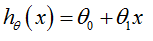### Understanding Basics of Machine Learning - ML Algo, Linear Regression, Cost Function, Gradient Descent

We will cover the following Machine Learning Basics:

## The two basic definitions of machine learning

1st by Arthur Samuel(1959)- Machine Learning: is Field of study that gives computers the ability to learn without being explicitly programmed.

2nd definition by Tom Mitchell (1998)- A computer program is said to learn from experience E with respect to some task T and some performance measure P, if its performance on T, as measured by P, improves with experience E.

## Machine Learning Algorithms: Supervised and unsupervised Learning

Supervised Learning is when the right answers (output/ target variables) are also given along with features(input variables) in the training data as input. In supervised learning we have Regression and Classification. Regression is when our output is continuous valued and Classification is when output is discrete valued.

Unsupervised Learning is when the right answers (output/ target variables) are not given. Clustering is an example of unsupervised learning. social network analysis, market segmentation, astronomical data analysis, organize computing clusters come under clustering.

## Linear Regression with one variable

First tried to make model for representing the linear regression with one variable taking an example of housing price prediction. In this example size (x) of house is taken as input variable/ feature. The price (y) is taken as the output/ target variable. The learning Algorithm for this problem is trained with training set. After training we get a hypothesis h which takes the size of house as input and gives the estimated price as output.

Representation of h
Where,
θand θ0 are parameters.

How to choose θi's : For this we have to study about Cost Function.

## Cost Function

Where,
θand θ2  are parameters.
Summation run for all training data.
m is total no of rows (examples) in training set.

Hypothesis:To determine θand θ2  our goal is to minimize J.

To minimize J we take partial derivatives of J w.r.t  θand θ2   and equate to zero and solve we will get formulae to get values of θand θ2   ---- this will give Normal Equation.

Other Method to determine is Gradient Descent Method

Here α is Learning Rate. If α is too small, gradient descent can be slow. And if α is too large, gradient descent can overshoot the minimum. It may fail to converge or even diverge.

Procedure:
• Keep changing θand θ2 to reduce J until we hope fully reach at minimum.

## Linear Regression with Multiple Variables (Multivariate Linear Regression)

n- total no of features,
m-total training examples,
xj -input(feature).

Also cover feature scaling.Idea of feature scaling is to make all features on a similar scale. One of the best method of feature scaling is Mean Normalization. We generally declare convergence if J( ) decreases by less than 10-3.

The other Method to solve for Multivariate Linear Regression is Normal Equation. It is generally recommended to use if no of features is less than 1000 but if it is larger value then gradient descent is more useful.